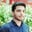Trusted answers to developer questions
Trusted Answers to Developer Questions

Related Tags

python
pandas
communitycreator

# What is Python pivot_table() in Pandas?Salman Yousaf

Grokking Modern System Design Interview for Engineers & Managers

Ace your System Design Interview and take your career to the next level. Learn to handle the design of applications like Netflix, Quora, Facebook, Uber, and many more in a 45-min interview. Learn the RESHADED framework for architecting web-scale applications by determining requirements, constraints, and assumptions before diving into a step-by-step design process.## Overview

pivot_table() creates a spreadsheet-style pivot table as a DataFrame in Pandas. The levels of the pivot table are stored in multiIndex objects, i.e., hierarchical indexes on the index and columns of the resultant DataFrame.

## Syntax

pandas.pivot_table(data,
values=None,
index=None,
columns=None,
aggfunc=’mean’,
fill_value=None,
margins=False,
dropna=True,
margins_name='All',
observed=False)

### Parameters

• data: This parameter represents the DataFrame.
• values: This is the column to aggregate. It is an optional parameter.
• index:
• Type = column, grouper, array, or list of the previous
• It is a required parameter. This parameter helps group our data using the index of the pivot table. When an array is passed, it must be used the same as the column values.
• columns:
• Type = column, grouper, array, or list of the previous
• It is a required parameter. This parameter helps group our data using the column of the pivot table. When an array is passed, it must be used the same as the column values.
Note: For index and columns parameter, if the array is passed, its length must be the same as the length of data. The list can contain any type other than the list.
• aggfunc:
• Type: A function, list of functions, or a dict
• Default: numpy.mean
• It is a required parameter.
Note: If we pass the list of functions, the resultant pivot table contains hierarchical columns. The top level of these columns are the names of functions. These names are concluded from the objects of functions by themselves. If we pass dict, the key is the column to aggregate. The value is a function or list of functions.
• fill_value:
• Type: Scalar
• Default = None
• It contains the value used to replace the missing values within the resultant pivot table after the aggregation.
• margins:
• Type: Boolean
• Default = False
• It is used to add all the columns and rows. For example, we can use it for grand totals and subtotals.
• dropna:
• Type: Boolean
• Default: True
• This parameter helps to exclude columns whose values are
• margins_name:
• Type: String
• Default: 'All'
• It is the name of the row or column that will hold the totals when the margins’ value is True.
• observed:
• Type: Boolean
• Default: False
• It applies if any of the groupers are categorical.
• If it is True, it displays the observed values for categorical grouper. If it is False, it displays all the values for categorical groupers.
• sort:
• Type: Boolean
• Default: True
• It must be defined to sort the result.

### Return value

pivot_table() returns the DataFrame, which is an Excel style pivot table.

### Code example

Let us discuss an example regarding the pivot_table() method.

import pandas as pdimport numpy as np dtf = pd.DataFrame({"cl1": ["pr1", "pr1", "pr1", "pr1", "pr1",                         "pr2", "pr2", "pr2", "pr2"],                   "cl2": ["1", "1", "1", "2", "2",                         "1", "1", "2", "2"],                   "cl3": ["half", "half", "half", "half",                         "full", "full", "half", "half",                         "full"],                   "cl4": [6, 3, 2, 6, 3, 2, 5, 1, 7],                   "cl5": [3, 4, 5, 2, 6, 5, 8, 8, 5]})print(dtf)# In the above code, we defined the dataframe.tbl1 = pd.pivot_table(dtf, values='cl4', index=['cl1', 'cl2'], columns=['cl3'], aggfunc=np.sum)print(tbl1)tbl2 = pd.pivot_table(dtf, values='cl4', index=['cl1', 'cl2'], columns=['cl3'], aggfunc=np.sum, fill_value=0)print(tbl2)tbl3 = pd.pivot_table(dtf, values=['cl4', 'cl5'], index=['cl1', 'cl3'], aggfunc={'cl4': np.mean,'cl5': np.mean})print(tbl3)tbl4 = pd.pivot_table(dtf, values=['cl4', 'cl5'], index=['cl1', 'cl3'], aggfunc={'cl4': np.mean,'cl5': [min, max, np.mean]})print(tbl4)
Using the pivot_table() method

### Explanation

• Line 13: We show the code to aggregate the values by calculating the sum.
• Line 15: We use the fill_value parameter to fill the missing value.
• Line 17:  We aggregate the values by calculating the mean across multiple columns.
• Line 19: We calculate multiple types of aggregation for any given value column.

RELATED TAGS

python
pandas
communitycreator

CONTRIBUTORSalman Yousaf

Grokking Modern System Design Interview for Engineers & Managers

Ace your System Design Interview and take your career to the next level. Learn to handle the design of applications like Netflix, Quora, Facebook, Uber, and many more in a 45-min interview. Learn the RESHADED framework for architecting web-scale applications by determining requirements, constraints, and assumptions before diving into a step-by-step design process.Keep Exploring

Learn in-demand tech skills in half the time